#### Final exam

The final exam is graded. We still recheck all addition and transcript. The average score was 82 when scaled to 100, the standard deviation 10. We had a global review on Wednesday, December 7, 2:30-4 and Thursday, December 8, 3:30-5, both in hall C. The final exam takes place on Monday, December 12, 2011 at 2 PM. The exam rooms were as follows:
```Last Name (A-M)  Emerson 105
Last Name (N-Z)  Sever   113
```
Check the Final exam schedule for other courses.Practice I, [Solutions]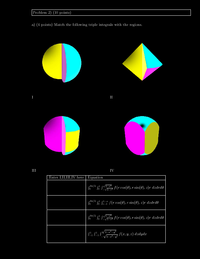Practice II, [Solutions]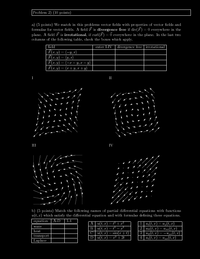Practice III, [Solutions]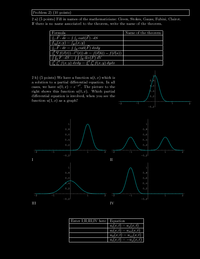Practice IV, [Solutions]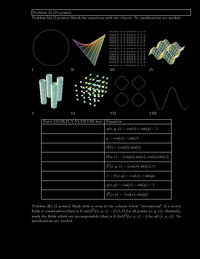Practice V, [Solutions]Practice VI, [Solutions]Practice VII, [Solutions]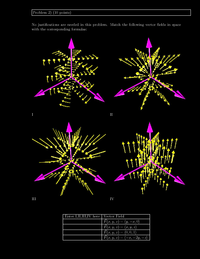Practice VIII, [Solutions]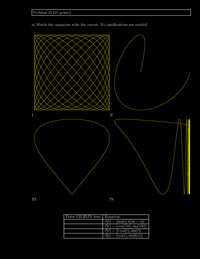Practice IX, [Solutions]

## Corrections: (The actual documents are always updated):

• Practice 1 (Corrected 12/6/2011 at noon): The thorn problem had some formula hidden behind the thorn.
• Practice 2 (Corrected 12/6/2011 at noon): d=18 instead of y=18 in solution of TF 13 Thanks to Jenny Shih
• Practice 1 (Corrected 12/6/2011 at 3 PM): Typo in 4) vector connecting points is <1,1,3>. Result is unchanged. Thanks to Daniel Backman.
• Practice 1 (corrected 12/9/2011 8 AM): Practice 1, problem 11, f(x,y,z)=sin(x) + 2y + sin(y) + exp(x), final answer does not change. In Problem 13, sin(phi) in the first integral is phi. Thanks to Bob Wu.
• Practice 2 (Corrected 12/10/2011 at 12 AM) Two typos in solutions to 9. Practice 8, problem 4, typo in a formula. Final result unchanged. Thanks to Sam Kim.
• Practice 4 (corrected 12/12/2011 at 6 AM) Typo in solution to problem 6. Values of function at global maxima. Thanks to Jonathan Miller

#### Second hourly

The second hourly took take place on Tuesday, November 1, 2011 in HALL C from 5:30 - 7PM. Note that the time is earlier than in in the first midterm. We had a global review on Sunday, October 30, 2011 from 7-8:30 in Lecture Hall D. The average of the exam was 84.5, the standard deviation 11.5.

Corrections: November 2-3: 7a,b). Thanks to Josh, Widly, William, Kuo-Kai for corrections.

Here were some practice exams for this test:Practice I, [Solutions]Practice II, [Solutions]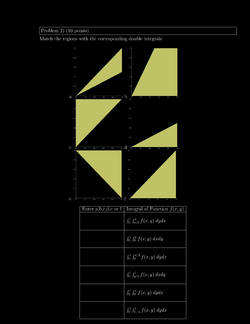Practice III, [Solutions]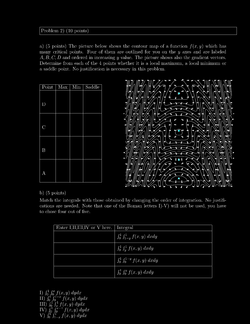Practice IV, [Solutions]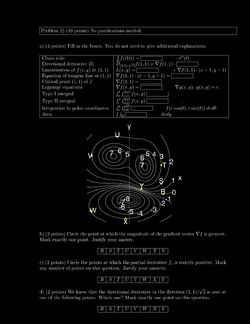Practice V, [Solutions]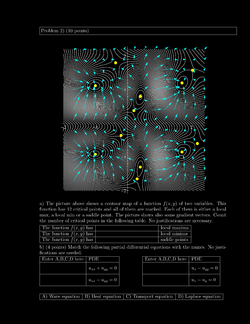Practice VI, [Solutions]

Errata:
• Corrected, October 24, 2011: Practice 1, Problem 6, the gradient is <0,-1,1> . The end result is correct. Thanks to Ben Sprung-Keyser. Several students have also mentioned that there was an inconsistency in nomenclature. Which is actually crucial: in order to find the tangent plane to a graph z=f(x,y), we introduce the function g(x,y,z)=f(x,y)-z and then take the gradient of this, which is = which is in our case <0,1,-1>. When knowing the gradient, it is easy to write down the plane ax+by+cz=d because d can be found by plugging in the point in ax+by+cz.

#### First hourly

The first exam took place on October 4, 2011 in Hall C from 7:00 to 8:30 PM . There was a global review on Sunday October 2, night from 7 to 9 PM in Lecture Hall D. The exam had an average score of 79 and a standard deviation of 11. Here is the exam with solutions. By the way, the name "hourly" is Harvard jargon which origins from times, when midterm exams were an hour long. Here were some practice exams for this test: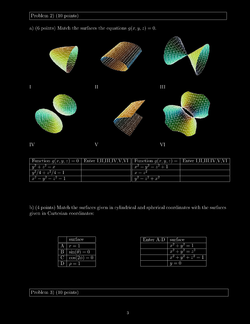Practice I, [Solutions]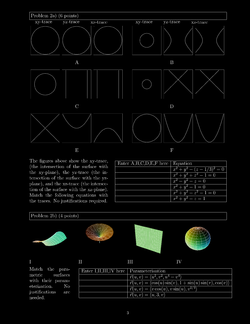Practice II, [Solutions]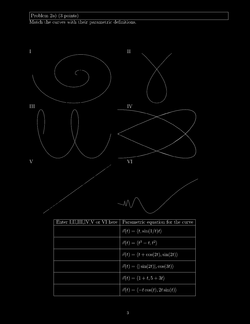Practice III, [Solutions]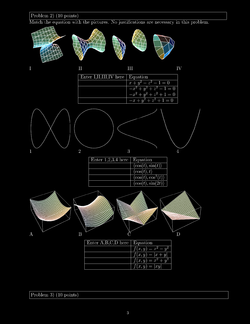Practice IV, [Solutions]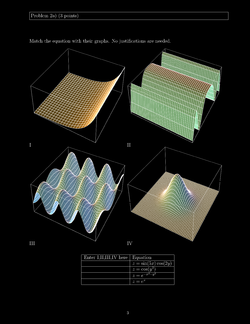Practice IV, [Solutions]Practice IV, [Solutions]

Errata:
• Corrected September 28, 12 AM: Practice 1, Problem 10b) cos(v) instead of sin(v) in z coordinate, 6a), typo: 3 instead of 13. Thanks to Ari Brenner
• Corrected September 30, 10:30 AM: Practice 1: 10b) sin(u) sin(v), Practice 2: 9b) 4t instead of 8t, Practice 4: 9b) coefficients lack sqrt(2). Thanks to Annie Yang.
• Corrected October 1, 10 AM, Practice 1, Comma missing in (u,v,sin(u v). Thanks to Sam Kim
• Corrected October 1, 9 PM, Practice 5, Problem 7a, 0 instead of 2 in acceleration. Thanks to Jenni Ting.